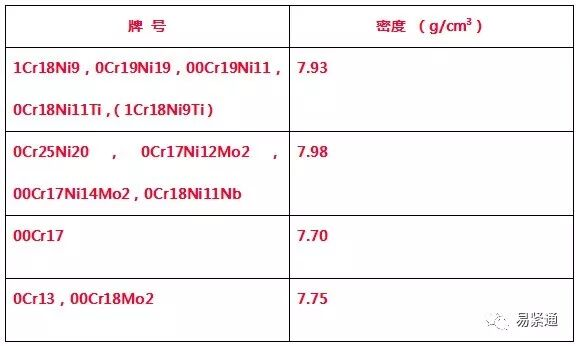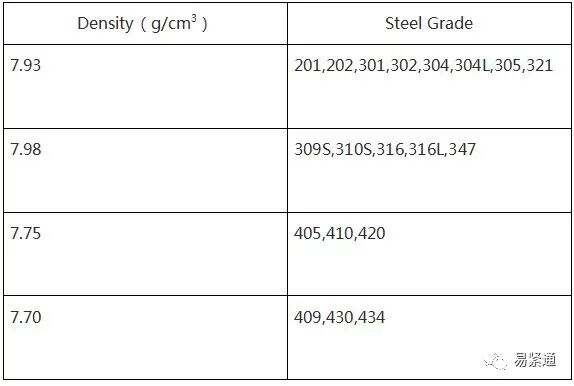• <samp id="8mweg"><sup id="8mweg"></sup></samp>
• <samp id="8mweg"><sup id="8mweg"></sup></samp>
• <input id="8mweg"><object id="8mweg"></object></input>
• <input id="8mweg"></input>

江西盛南五金有限公司歡迎您的光臨！資訊中心 News Center
聯系人：溫小姐 (女士)
手機：+86-13777787565
電話：0577-86666224
郵箱：wendy@wzsnjgj.com
地址：江西省上饒市鄱陽縣工業園區蘆田輕工產業基地
新聞資訊
您所在的位置：首頁 > 新聞資訊
常用金屬重量計算公式，留著總有用處
更新時間：2018-10-09

圓鋼重量（公斤）=0.00617×直徑×直徑×長度

方鋼重量（公斤）=0.00785×邊寬×邊寬×長度

六角鋼重量（公斤）=0.0068×對邊寬×對邊寬×長度

八角鋼重量（公斤）=0.0065×對邊寬×對邊寬×長度

螺紋鋼重量（公斤）=0.00617×計算直徑×計算直徑×長度

角鋼重量（公斤）=0.00785×（邊寬+邊寬-邊厚）×邊厚×長度

扁鋼重量（公斤）=0.00785×厚度×邊寬×長度

鋼管重量（公斤）=0.02466×壁厚×（外徑-壁厚）×長度

鋼板重量（公斤）=7.85×厚度×面積

圓紫銅棒重量（公斤）=0.00698×直徑×直徑×長度

圓黃銅棒重量（公斤）=0.00668×直徑×直徑×長度

圓鋁棒重量（公斤）=0.0022×直徑×直徑×長度

方紫銅棒重量（公斤）=0.0089×邊寬×邊寬×長度

方黃銅棒重量（公斤）=0.0085×邊寬×邊寬×長度

方鋁棒重量（公斤）=0.0028×邊寬×邊寬×長度

六角紫銅棒重量（公斤）=0.0077×對邊寬×對邊寬×長度

六角黃銅棒重量（公斤）=0.00736×邊寬×對邊寬×長度

六角鋁棒重量（公斤）=0.00242×對邊寬×對邊寬×長度

紫銅板重量（公斤）=0.0089×厚×寬×長度

黃銅板重量（公斤）=0.0085×厚×寬×長度

鋁板重量（公斤）=0.00171×厚×寬×長度

圓紫銅管重量（公斤）=0.028×壁厚×（外徑-壁厚）×長度

圓黃銅管重量（公斤）=0.0267×壁厚×（外徑-壁厚）×長度

圓鋁管重量（公斤）=0.00879×壁厚×（外徑-壁厚）×長度

注：公式中長度單位為米，面積單位為平方米，其余單位均為毫米

以上重量X材料單價為材料費.

加上表面處理+每個工藝流程的工時費+包裝材料+出貨費+稅金+利率= 報價(FOB)

關于絲網類計算的幾個公式及參數

求絲網的重量：

絲直徑x絲直徑x目數=1平方米的（市斤）重量

求鋼板網的重量：

1平方米鋼板的重量÷延長率=1平方米鋼板網的重量

求鋼板的重量：

板厚x比重=1平方米鋼板的重量

常用材料的比重：

鐵=7.85 鋁=2.7 銅=8.95 不銹鋼=7.93

求鋼板網的延長率：

鋼板網菱形孔短方向節距÷2倍的梗=1平方米鋼板能拉伸到幾米鋼板網

求鐵絲的重量：

絲直徑x絲直徑x0.00617=1米鐵絲的重量

304不銹鋼的密度

計算公式：W=3.1416×壁厚（外徑- 壁厚）×密度

式中，W單位kg/m; 壁厚、外徑單位mm; 密度單位見下表。

流體輸送用不銹鋼焊接鋼管的密度理論重量計算公式：Calculation of Theoretic Weight

鋼品理論重量是Theoretic Weight

重量（kg）=厚度（mm）*寬度（m）*長度（m）*密度值

Weight（kg）=Thickness（mm）*Width（m）*Length（m）*Density（g/cm3是以kg/cm3為單位。

以上是幾種比較常用的不銹鋼密度表,僅供參考.如果你只是概算，可按一般鋼鐵密度7850kg/m3計算．

不銹鋼重量簡易計算公式

不銹鋼平板每平方米重量(kg)計算公式：比重*厚度(mm)*寬度(mm)*長度(m)

304、321管每米重量(kg)計算公式:0.02491*壁厚(mm)*(外徑-壁厚)(mm)

316L、310S管每米重量(kg)計算公式：0.02495*壁厚(mm)*(外徑-壁厚)(mm)

不銹元鋼每米重量(kg)計算公式：直徑(mm)*直徑(mm)* (鎳不銹:0.00623；鉻不銹:0.00609)

鋼材理論重量計算

鋼材理論重量計算的計量單位為公斤（kg）。其基本公式為：

W（重量，kg ）=F(斷面積 mm2)×L(長度，m)×ρ(密度，g/cm3)×1/1000

各種鋼材理論重量計算公式如下：

圓鋼 盤條（kg/m）

W= 0.006165 ×d×d

d = 直徑mm

直徑100 mm 的圓鋼，求每m 重量。每m 重量= 0.006165 ×1002=61.65kg

螺紋鋼（kg/m）

W= 0.00617 ×d×d

d= 斷面直徑mm

斷面直徑為12 mm 的螺紋鋼，求每m 重量。每m 重量=0.00617 ×12 2=0.89kg

方鋼（kg/m）

W= 0.00785 ×a ×a

a= 邊寬mm

邊寬20 mm 的方鋼，求每m 重量。每m 重量= 0.00785 ×202=3.14kg

扁鋼 （kg/m）

W= 0.00785 ×b ×d

b= 邊寬mm

d= 厚mm

邊寬40 mm ，厚5mm 的扁鋼，求每m 重量。每m 重量= 0.00785 ×40 ×5= 1.57kg

六角鋼 （kg/m）

W= 0.006798 ×s×s

s= 對邊距離mm

對邊距離50 mm 的六角鋼，求每m 重量。每m 重量= 0.006798 ×502=17kg

八角鋼 （kg/m）

W= 0.0065 ×s ×s

s= 對邊距離mm

對邊距離80 mm 的八角鋼，求每m 重量。每m 重量= 0.0065 ×802=41.62kg

等邊角鋼 （kg/m）

= 0.00785 ×[d （2b – d ）+0.215 （R2 – 2r2 ）]

b= 邊寬

d= 邊厚

R= 內弧半徑

r= 端弧半徑

求20 mm ×4mm 等邊角鋼的每m 重量。從冶金產品目錄中查出4mm ×20 mm 等邊角鋼的R為3.5 ，r 為1.2 ，則每m 重量= 0.00785 ×[4 ×（2 ×20 – 4 ）+0.215 ×（3.52 – 2×1.2 2）]=1.15kg

不等邊角鋼 （kg/m）

W= 0.00785 ×[d （B+b – d ）+0.215 （R2 – 2 r 2 ）]

B= 長邊寬

b= 短邊寬

d= 邊厚

R= 內弧半徑

r= 端弧半徑

求30mm×20mm×4mm 不等邊角鋼的每m 重量。從冶金產品目錄中查出30×20×4 不等邊角鋼的R 為3.5，r 為1.2，則每m 重量=0.00785 ×[4×（30+20–4）+0.215×（3.52–2×1.22）]=1.46kg

槽鋼 （kg/m）

W=0.00785 ×[hd+2t（b–d）+0.349（R2–r2 ）]

h= 高

b= 腿長

d= 腰厚

t= 平均腿厚

R= 內弧半徑

r= 端弧半徑

求80 mm ×43mm ×5mm 的槽鋼的每m 重量。從冶金產品目錄中查出該槽鋼t為8，R為8，r為4，則每m重量=0.00785 ×[80×5+2×8 ×（43–5）+0.349×（82–4 2）]=8.04kg

工字鋼（kg/m）

W=0.00785×[hd+2t（b–d ）+0.615（R2–r2 ）]

h= 高

b= 腿長

d= 腰厚

t= 平均腿厚

R= 內弧半徑

r= 端弧半徑

求250 mm ×118mm×10mm 的工字鋼每m 重量。從金屬材料手冊中查出該工字鋼t 為13，R為10，r 為5，則每m 重量= 0.00785×[250×10+2×13×（118–10 ）+0.615×（102–52）]=42.03kg

鋼板（kg/m2 ）

W= 7.85 ×d

d= 厚

厚度 4mm 的鋼板，求每m2 重量。每m2 重量=7.85 ×4=31.4kg

鋼管（包括無縫鋼管及焊接鋼管（kg/m）

W=0.02466×S（D–S ）

D= 外徑

S= 壁厚

外徑為60 mm 壁厚4mm 的無縫鋼管，求每m重量。每m 重量=0.02466×4×（60–4）=5.52kg

一级欧美片一级日韩片,澳门一级毛片免费播放,白天干了晚上接着干的成语
• <samp id="8mweg"><sup id="8mweg"></sup></samp>
• <samp id="8mweg"><sup id="8mweg"></sup></samp>
• <input id="8mweg"><object id="8mweg"></object></input>
• <input id="8mweg"></input>